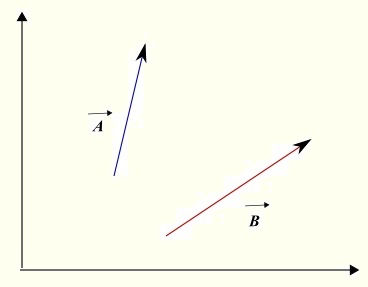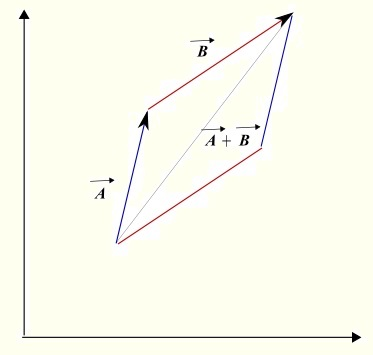# Addition and Subtraction of Vectors

 Addition and Subtraction of Vectors Figure 1, below, shows two vectors on a plane. To add the two vectors, translate one of the vectors so that the terminal point of one vector coincides with the starting point of the second vector and the sum is a vector whose starting point is the starting point of the first vector and the terminal point is the terminal point of the second vector as shown in figure 2.Fig1. - 2 vectors in 2 dimensions.Fig2. - Add 2 vectors in 2 dimensions - Parallelogram. When the components of the two vectors are known, the sum of two vectors is found by adding corresponding components. Example 1 Given vectors A = (2 , -4) and B = (4 , 8), what are the components of A → + B→ Solution A → + B→ = (2 ,-4 ) + (4 , 8) = (2 + 4 ,-4 + 8 ) = (6 , 4) The subtraction of two vectors is shown in figure 3. The idea is to change the subtraction into an addition as follows: A → - B→ = A → + (-B)→Fig3. - subtract 2 vectors. Example 2 The magnitudes of two vectors U and V are equal to 5 and 8 respectively. Vector U makes an angle of 20� with the positive direction of the x-axis and vector V makes an angle of 80� with the positive direction of the x-axis. Both angles are measured counterclockwise. Find the magnitudes and directions of vectors U + V and U - V. Solution Let us first use the magnitudes and directions to find the components of vectors U and V. U → = (5 cos(20�) , 5 sin(20�)) V → = (10 cos(80�) , 10 sin(80�)) Magnitude and direction of vector U + V U → + V→ = (5 cos(20�) , 5 sin(20�)) + (10 cos(80�) , 10 sin(80�)) = (5 cos(20�) + 10 cos(80�) , 5 sin(20�)+10 sin(80�)) Now that we have the components of vector U + V, we can calculate the magnitude as follows: | U → + V→| = √ (5 cos(20�) + 10 cos(80�)) 2 + (5 sin(20�)+10 sin(80�)) 2 = 5√7 ≈ 13.22 If θ is the angle in standard position (angle between vector U+V and x-axis positive direction) of vector U + V, then tan(θ) = y-component of U+V / x-component of U+V = 5 sin(20�)+10 sin(80�) / 5 cos(20�) + 10 cos(80�) The reference angle α to angle θ is given by α = arctan|( 5 sin(20�)+10 sin(80�) / 5 cos(20�) + 10 cos(80�) )| ≈ 60.9� We now approximate the components of vector U + V so that we can determine the quadrant of U + V U → + V→ = (5 cos(20�) + 10 cos(80�) , 5 sin(20�)+10 sin(80�)) ≈ (6.43 , 11.6) Since both components of vector U + V are positive, the terminal side of angle θ is in quadrant I and therefore θ = α = 60.9� The direction of vector U + V is given by an angle approximately equal to 60.9�. This angle is measured in counterclockwise direction from the positive x-axis. Magnitude and direction of vector U - V U → - V→ = (5 cos(20�) , 5 sin(20�)) - (10 cos(80�) , 10 sin(80�)) = (5 cos(20�) - 10 cos(80�) , 5 sin(20�) - 10 sin(80�)) Now that we have the components of vector U - V, we can calculate the magnitude as follows: | U → - V→| = √ (5 cos(20�) - 10 cos(80�)) 2 + (5 sin(20�) - 10 sin(80�)) 2 = 5√3 ≈ 8.66 If β is the angle in standard position (angle between vector U - V and x-axis positive direction) of vector U - V, then tan(β) = y-component of U-V / x-component of U-V = 5 sin(20�) - 10 sin(80�) / 5 cos(20�) - 10 cos(80�) The reference angle α to angle β is given by α = arctan|( 5 sin(20�) - 10 sin(80�) / 5 cos(20�) - 10 cos(80�) )| = 70� We now approximate the components of vector U - V so that we can determine the quadrant of U - V U → - V→ = (5 cos(20�) - 10 cos(80�) , 5 sin(20�) - 10 sin(80�)) ≈ (2.96 , -8.13 ) The signs of the components of vector U - V indicate that terminal side of angle β is in quadrant IV and therefore β = 360� - α = 360� - 70� = 290� The direction of vector U - V is given by an angle equal to 290�. This angle is measured in counterclockwise direction from the positive x-axis. Example 3 The components of three vectors A, B and C are given as follows: A → = (2 , -1), B → = (-3 , 2) and C → = (13, - 8). Find real numbers a and b such that C → = a A → + b B →. Solution We first rewrite the equation C → = a A → + b B → using the components of the vectors. (13, - 8) = a (2 , -1) + b (-3 , 2) Rewrite an equation for each component 13 = 2 a - 3 b and - 8 = - a + 2 b Solve the above equations in a and b to obtain a = 2 and b = -3.